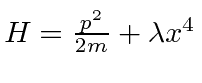## Homework Problems

1. Calculate the lowest order energy shift for the (0th order degenerate first) excited states of Heliumwhere. This problem is set up in the discussion of the first excited states. The following formulas will aid you in the computation. First, we can expand the formula for the inverse distance between the two electrons as follows.Hereis the smaller of the two radii andis the larger. As in the ground state calculation, we can use the symmetry of the problem to specify which radius is the larger. Then we can use a version of the addition theorem to write the Legendre Polynomialin terms of the spherical hamonics for each electron.Using the equation, this sets us up to do our integrals nicely.

2. Consider the lowest state of ortho-helium. What is the magnetic moment? That is what is the interaction with an external magnetic field?
3. A proton and neutron are bound together into a deuteron, the nucleus of an isotope of hydrogen. The binding energy is found to be -2.23 MeV for the nuclear ground state, anstate. Assuming a potential of the form, withFermis, use the variational principle to estimate the strength of the potential.

4. Use the variational principle with a gaussian trial wave function to prove that a one dimensional attractive potential will always have a bound state.

5. Use the variational principle to estimate the ground state energy of the anharmonic oscillator,.

Jim Branson 2013-04-22